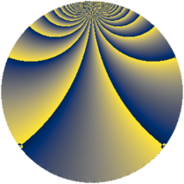# Properties

 Label 900.3.bcLevel $900$ Weight $3$ Character orbit 900.bc Rep. character $\chi_{900}(157,\cdot)$ Character field $\Q(\zeta_{12})$ Dimension $144$ Sturm bound $540$

# Related objects

## Defining parameters

 Level: $$N$$ $$=$$ $$900 = 2^{2} \cdot 3^{2} \cdot 5^{2}$$ Weight: $$k$$ $$=$$ $$3$$ Character orbit: $$[\chi]$$ $$=$$ 900.bc (of order $$12$$ and degree $$4$$) Character conductor: $$\operatorname{cond}(\chi)$$ $$=$$ $$45$$ Character field: $$\Q(\zeta_{12})$$ Sturm bound: $$540$$

## Dimensions

The following table gives the dimensions of various subspaces of $$M_{3}(900, [\chi])$$.

Total New Old
Modular forms 1512 144 1368
Cusp forms 1368 144 1224
Eisenstein series 144 0 144

## Trace form

 $$144q + 2q^{3} + O(q^{10})$$ $$144q + 2q^{3} - 24q^{11} - 36q^{17} - 104q^{21} + 12q^{23} - 46q^{27} + 14q^{33} + 84q^{37} - 144q^{41} + 78q^{47} + 248q^{51} + 312q^{53} + 338q^{57} + 192q^{61} + 242q^{63} + 78q^{67} + 264q^{71} + 240q^{77} + 1148q^{81} - 132q^{83} - 172q^{87} - 336q^{91} - 364q^{93} + 90q^{97} + O(q^{100})$$

## Decomposition of $$S_{3}^{\mathrm{new}}(900, [\chi])$$ into newform subspaces

The newforms in this space have not yet been added to the LMFDB.

## Decomposition of $$S_{3}^{\mathrm{old}}(900, [\chi])$$ into lower level spaces

$$S_{3}^{\mathrm{old}}(900, [\chi]) \cong$$ $$S_{3}^{\mathrm{new}}(45, [\chi])$$$$^{\oplus 6}$$$$\oplus$$$$S_{3}^{\mathrm{new}}(90, [\chi])$$$$^{\oplus 4}$$$$\oplus$$$$S_{3}^{\mathrm{new}}(180, [\chi])$$$$^{\oplus 2}$$$$\oplus$$$$S_{3}^{\mathrm{new}}(225, [\chi])$$$$^{\oplus 3}$$$$\oplus$$$$S_{3}^{\mathrm{new}}(450, [\chi])$$$$^{\oplus 2}$$